Courses

# Projectile Motion (Part - 2) - Physics, Solution by DC Pandey NEET Notes | EduRev

## DC Pandey (Questions & Solutions) of Physics: NEET

Created by: Ciel Knowledge

## NEET : Projectile Motion (Part - 2) - Physics, Solution by DC Pandey NEET Notes | EduRev

The document Projectile Motion (Part - 2) - Physics, Solution by DC Pandey NEET Notes | EduRev is a part of the NEET Course DC Pandey (Questions & Solutions) of Physics: NEET.
All you need of NEET at this link: NEET

Introductory Exercise 4.2

Ques 1: A particle is projected along an inclined plane as shown in figure. What is the speed of the particle when it collides at point A? (g = 10 m/s2)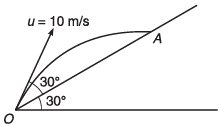Ans: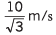Sol: Time of flight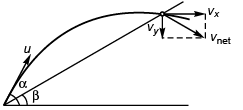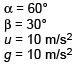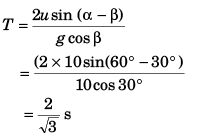Using,  v = u + at
vx = ux = u cos 60°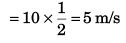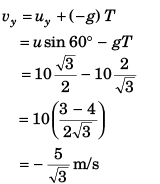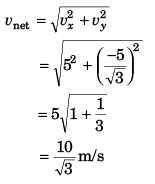Ques 2: In the above problem what is the component of its velocity perpendicular to the plane when it strikes at A?
Ans: 5 m/s
Sol: Component of velocity perpendicular to plane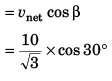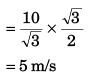Ques 3: Two particles A and B are projected simultaneously from the two towers of height 10 m and 20 m respectively. Particle A is projected with an initial speed of 10√2 m/s at an angle of 45° with horizontal, while particle B is projected horizontally with speed 10 m/s. If they collide in air, what is the distance d between the towers?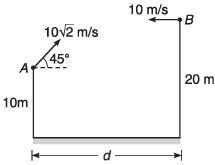Ans: 20 m
Sol: Let the particle collide at time t.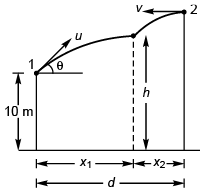x1 = (u cos θ) t
and x2 = vt
∴ d = x2 - x1
= (v + u cos θ) t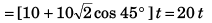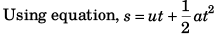For vertical motion of particle 1: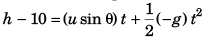i.e.,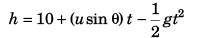…(i)
or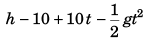For the vertical motion of particle 2: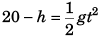i.e.,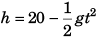…(ii)
Comparing Eqs. (i) and (ii),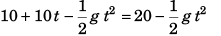⇒ t = 1 s
∴ d = 20 m

Ques 4: Two particles A and B are projected from ground towards each other with speeds 10 m/s and 5√2 m/s at angles 30° and 45° with horizontal from two points separated by a distance of 15 m. Will they collide or not?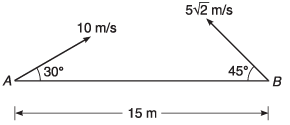Ans: No
Sol: u = 10 m/s
v = 5√2 m/s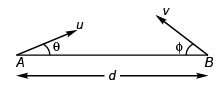θ = 30°
φ = 45°
d = 15 m
Let the particles meet (or are in the same vertical time t).
∴ d = (u cos θ) t + (v cos φ) t
⇒ 15 = (10 cos 30° + 5√2 cos 45°) t
or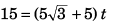or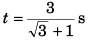= 1.009 s
Now, let us find time of flight of A and B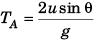= 1 s
As TA < t, particle A will touch ground before the expected time t of collision.
∴ Ans: NO.

Ques 5: A particle is projected from the bottom of an inclined plane of inclination 30°. At what angle α (from the horizontal) should the particle be projected to get the maximum range on the inclined plane.
Ans: 60°
Sol: For range to be maximum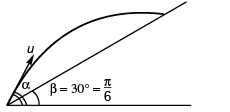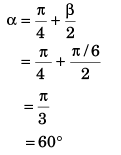Ques 6: A particle is projected from the bottom of an inclined plane of inclination 30° with velocity of 40 m/s at an angle of 60° with horizontal. Find the speed of the particle when its velocity vector is parallel to the plane. Take g = 10 m/s2.
Ans: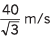Sol: At point A velocity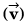of the particle will be parallel to the inclined plane.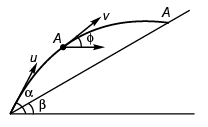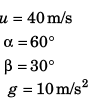∴ φ = β
vx = ux = u cos α
vx = v cos φ = v cos β
or u cos α = v cos β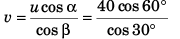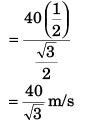Ques 7: Two particles A and B are projected simultaneously in the directions shown in figure with velocities vA = 20 m/s and vB = 10 m/s respectively. They collide in air after 1/2 s.
Find:
(a) the angle θ
(b) the distance x.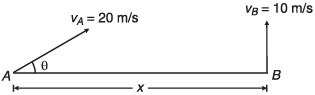Ans: (a) 30°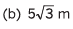Sol: (a) At time t, vertical displacement of A
= Vertical displacement of B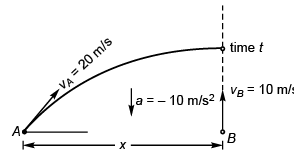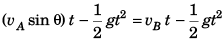i.e.,  vA sin θ = vB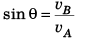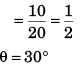∴ θ = 30°
(b) x = (vA cos θ) t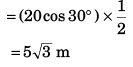Offer running on EduRev: Apply code STAYHOME200 to get INR 200 off on our premium plan EduRev Infinity!

210 docs

,

,

,

,

,

,

,

,

,

,

,

,

,

,

,

,

,

,

,

,

,

,

,

,

;